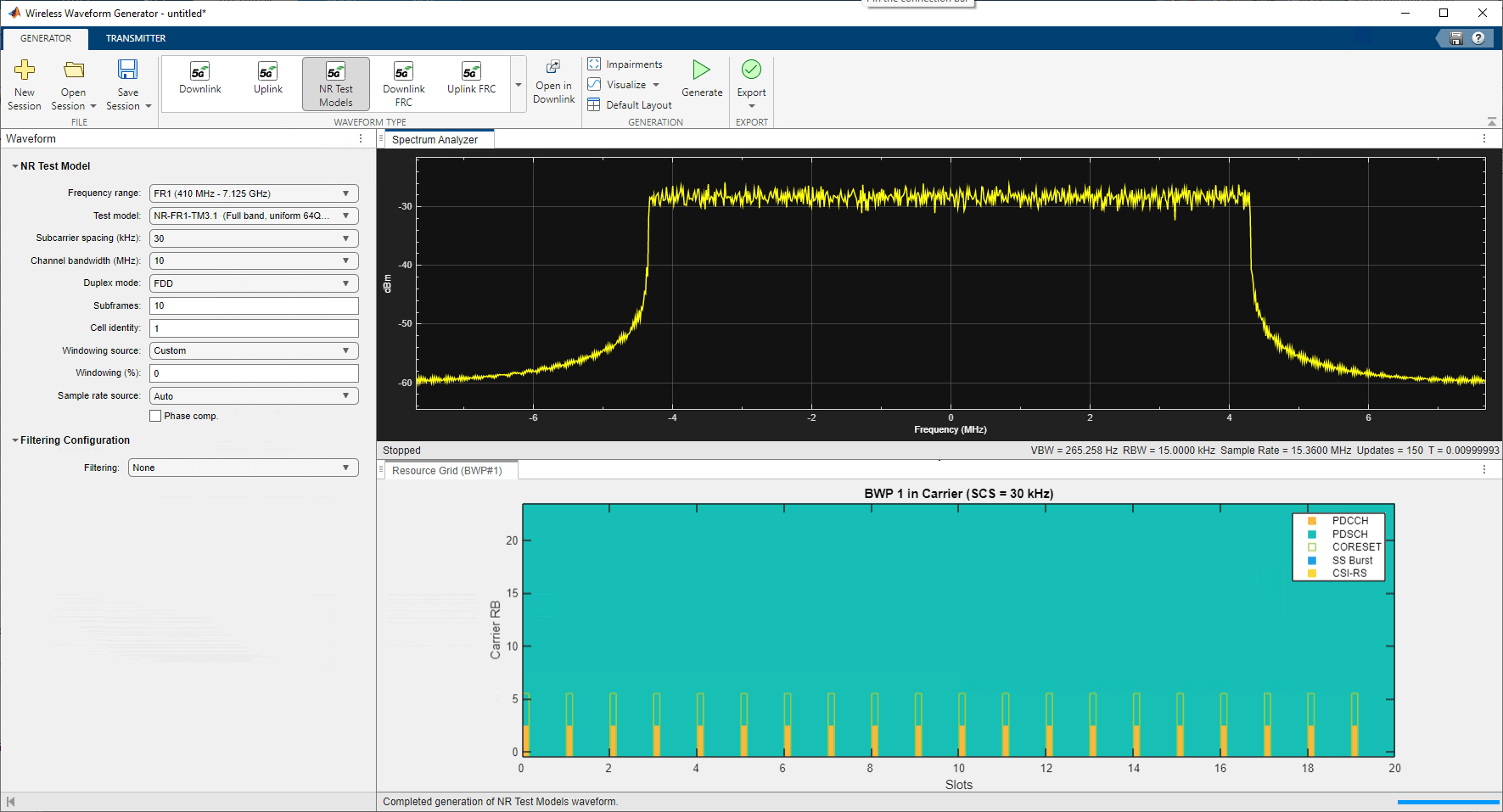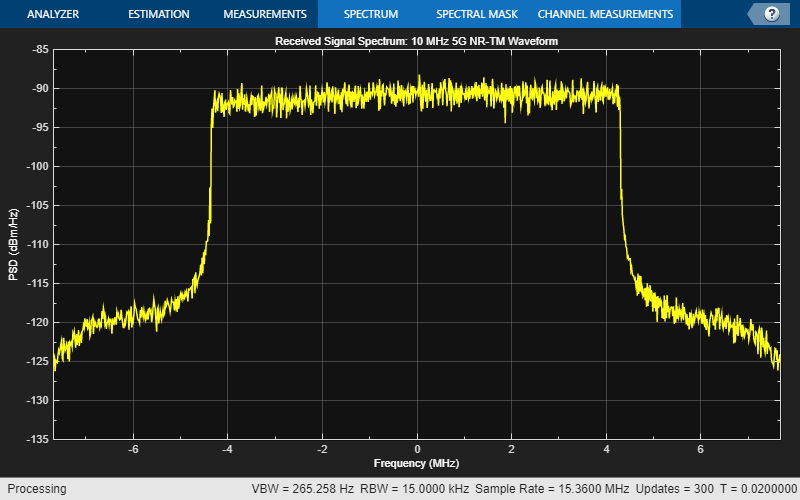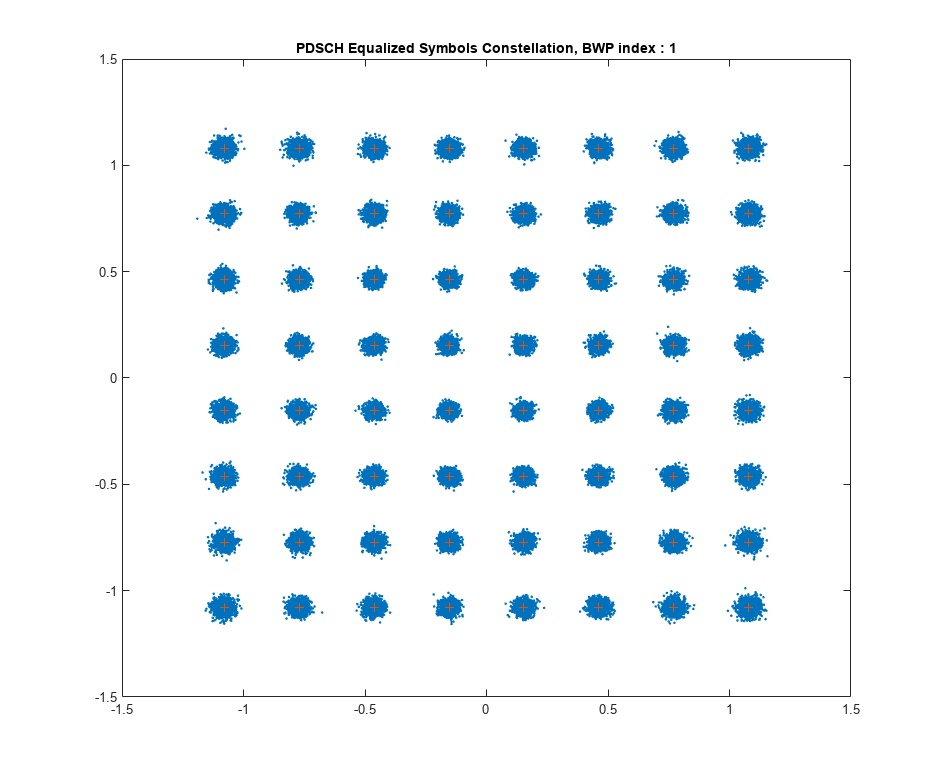# 5G NR Waveform Acquisition and Analysis

This example shows how to generate a 5G NR test model (NR-TM) waveform using the 5G Waveform Generator app and download the generated waveform to a Keysight™ vector signal generator for over-the-air transmission using the Instrument Control Toolbox™ software. The example then captures the transmitted over-the-air signal using a Keysight signal analyzer and analyzes the signal in MATLAB®.

### Introduction

This example generates a 5G NR-TM waveform using the 5G Waveform Generator app, downloads and transmits the waveform onto a Keysight vector signal generator, and then receives the waveform using a Keysight signal analyzer for waveform analysis in MATLAB. This diagram shows the general workflow.Requirements

To run this example, you need these instruments:

• Keysight E4438C ESG vector signal generator

• Keysight N9030A PXA signal analyzer

### Generate Baseband Waveform Using 5G Waveform Generator App

In MATLAB, on the Apps tab, click the 5G Waveform Generator app.

In the Waveform Type section, click NR Test Models. In the left-most pane of the app, you can set the parameters for the selected waveform. For this example:

• Set Frequency range as `FR1 (410 MHz - 7.125 GHz)`

• Set Test model as `NR-FR1-TM3.1 (Full band, uniform 64 QAM)`

• Set Channel bandwidth (MHz) as `10`

• Set Subcarrier spacing (kHz) as `30`

• Set Duplex mode as `FDD`.

On the app toolstrip, click Generate.

```% Set the NR-TM parameters for the receiver nrtm = "NR-FR1-TM3.1"; % Reference channel bw = "10MHz"; % Channel bandwidth scs = "30kHz"; % Subcarrier spacing dm = "FDD"; % Duplexing mode```

This figure shows a 10 MHz 5G NR waveform visible at baseband.### Transmit Over-the-Air Signal

Download the generated signal to the RF signal generator over one of the supported communication interfaces (requires Instrument Control Toolbox). The app automatically finds the signal generator that is connected over the TCP/IP interface. On the Transmitter tab of the app, select `Agilent/Keysight Signal Generator SCPI` from the Driver list. Set the Center frequency (GHz) parameter to `3.4` and the Output power (dBm) parameter to `-15`. The app automatically obtains the baseband sample rate from the generated waveform. To start the transmission, click Transmit in the toolstrip.### Read IQ Data from a Signal Analyzer over TCP/IP

To read the in-phase and quadrature (IQ) data into MATLAB for analysis, configure the Keysight N9030A signal analyzer using the Instrument Control Toolbox software.

Define the instrument configuration parameters based on the signal you are measuring.

```% Set parameters for the spectrum analyzer centerFrequency = 3.4e9; sampleRate = 15.36e6; measurementTime = 20e-3; mechanicalAttenuation = 0; %dB startFrequency = 3.39e9; stopFrequency = 3.41e9; resolutionBandwidth = 220e3; videoBandwidth = 220000;```

Perform these steps before connecting to the spectrum analyzer.

• Find the resource ID of the Keysight N9030A signal analyzer.

• Connect to the instrument using the virtual instrument software architecture (VISA) interface.

• Adjust the input buffer size to hold the data that the instrument returns.

• Set the timeout to allow sufficient time for the measurement and data transfer.

```foundVISA = visadevlist; resourceID = foundVISA(foundVISA.Model == "N9030A",:).ResourceName; resourceID = resourceID(contains(resourceID,"N9030A")); % Extract resourceID which contains "N9030A" sigAnalyzerObj = visadev(resourceID); sigAnalyzerObj.ByteOrder = "big-endian"; configureCallback(sigAnalyzerObj,"byte",85e6,@callbackFcn) sigAnalyzerObj.Timeout = 20;```

Reset the instrument to a known state using the appropriate standard command for programmable instruments (SCPI). Query the instrument identity to ensure the correct instrument is connected.

```writeline(sigAnalyzerObj,"*RST"); instrumentInfo = writeread(sigAnalyzerObj,"*IDN?"); fprintf("Instrument identification information: %s",instrumentInfo);```
```Instrument identification information: Agilent Technologies,N9030A,US00071181,A.14.16 ```

The X-Series signal and spectrum analyzers perform IQ measurements as well as spectrum measurements. In this example, you acquire time domain IQ data, visualize the data using MATLAB, and perform signal analysis on the acquired data. The SCPI commands configure the instrument and define the format of the data transfer after the measurement is complete.

```% Set up signal analyzer mode to basic IQ mode writeline(sigAnalyzerObj,":INSTrument:SELect BASIC"); % Set the center frequency writeline(sigAnalyzerObj,strcat(":SENSe:FREQuency:CENTer ",num2str(centerFrequency))); % Set the capture sample rate writeline(sigAnalyzerObj,strcat(":SENSe:WAVeform:SRATe ",num2str(sampleRate))); % Turn off averaging writeline(sigAnalyzerObj,":SENSe:WAVeform:AVER OFF"); % Set the spectrum analyzer to take one single measurement after the trigger line goes high writeline(sigAnalyzerObj,":INIT:CONT OFF"); % Set the trigger to external source 1 with positive slope triggering writeline(sigAnalyzerObj,":TRIGger:WAVeform:SOURce IMMediate"); writeline(sigAnalyzerObj,":TRIGger:LINE:SLOPe POSitive"); % Set the time for which measurement needs to be made writeline(sigAnalyzerObj,strcat(":WAVeform:SWE:TIME ",num2str(measurementTime))); % Turn off electrical attenuation writeline(sigAnalyzerObj,":SENSe:POWer:RF:EATTenuation:STATe OFF"); % Set the mechanical attenuation level writeline(sigAnalyzerObj,strcat(":SENSe:POWer:RF:ATTenuation ",num2str(mechanicalAttenuation))); % Turn IQ signal ranging to auto writeline(sigAnalyzerObj,":SENSe:VOLTage:IQ:RANGe:AUTO ON"); % Set the endianness of returned data writeline(sigAnalyzerObj,":FORMat:BORDer NORMal"); % Set the format of the returned data writeline(sigAnalyzerObj,":FORMat:DATA REAL,64");```

Trigger the instrument to make the measurement. Wait for the measurement operation to complete, and then read-in the waveform. Before processing the data, separate the I and Q components from the interleaved data that is received from the instrument and create a complex vector in MATLAB.

```% Trigger the instrument and initiate measurement writeline(sigAnalyzerObj,"*TRG"); writeline(sigAnalyzerObj,":INITiate:WAVeform"); % Wait until measure operation is complete measureComplete = writeread(sigAnalyzerObj,"*OPC?"); % Read the IQ data writeline(sigAnalyzerObj,":READ:WAV0?"); data = readbinblock(sigAnalyzerObj,"double"); % Separate the data and build the complex IQ vector inphase = data(1:2:end); quadrature = data(2:2:end); rxWaveform = inphase+1i*quadrature;```

Capture and display the information about the most recently acquired data.

```writeline(sigAnalyzerObj,":FETCH:WAV1?"); signalSpec = readbinblock(sigAnalyzerObj,"double"); % Display the measurement information captureSampleRate = 1/signalSpec(1); fprintf("Sample Rate (Hz) = %s",num2str(captureSampleRate));```
```Sample Rate (Hz) = 15360000 ```
`fprintf("Number of points read = %s",num2str(signalSpec(4)));`
```Number of points read = 307201 ```
`fprintf("Max value of signal (dBm) = %s",num2str(signalSpec(6)));`
```Max value of signal (dBm) = -39.0019 ```
`fprintf("Min value of signal (dBm) = %s",num2str(signalSpec(7)));`
```Min value of signal (dBm) = -107.9755 ```

Plot the spectrum of the acquired waveform to confirm the bandwidth of the received signal.

```% Ensure rxWaveform is a column vector if ~iscolumn(rxWaveform) rxWaveform = rxWaveform.'; end % Plot the power spectral density (PSD) of the acquired signal spectrumPlotRx = dsp.SpectrumAnalyzer; spectrumPlotRx.SampleRate = captureSampleRate; spectrumPlotRx.SpectrumType = "Power density"; spectrumPlotRx.PowerUnits = "dBm"; spectrumPlotRx.Window = "Hamming"; spectrumPlotRx.SpectralAverages = 10; spectrumPlotRx.YLimits = [-140 -90]; spectrumPlotRx.YLabel = "PSD"; spectrumPlotRx.ShowLegend = false; spectrumPlotRx.Title = "Received Signal Spectrum: 10 MHz 5G NR-TM Waveform"; spectrumPlotRx(rxWaveform);```Switch the instrument to spectrum analyzer mode and compare the spectrum view generated in MATLAB with the view on the signal analyzer. Use additional SCPI commands to configure the instrument measurement and display settings.

```% Switch back to the spectrum analyzer view writeline(sigAnalyzerObj,":INSTrument:SELect SA"); % Set the mechanical attenuation level writeline(sigAnalyzerObj,strcat(":SENSe:POWer:RF:ATTenuation ",num2str(mechanicalAttenuation))); % Set the center frequency, RBW, and VBW writeline(sigAnalyzerObj,strcat(":SENSe:FREQuency:CENTer ",num2str(centerFrequency))); writeline(sigAnalyzerObj,strcat(":SENSe:FREQuency:STARt ",num2str(startFrequency))); writeline(sigAnalyzerObj,strcat(":SENSe:FREQuency:STOP ",num2str(stopFrequency))); writeline(sigAnalyzerObj,strcat(":SENSe:BANDwidth:RESolution ",num2str(resolutionBandwidth))); writeline(sigAnalyzerObj,strcat(":SENSe:BANDwidth:VIDeo ",num2str(videoBandwidth))); % Enable continuous measurement on the spectrum analyzer writeline(sigAnalyzerObj,":INIT:CONT ON"); % Begin receiving the over-the-air signal writeline(sigAnalyzerObj,"*TRG");```

For instrument cleanup, clear the instrument connection:

`clear sigAnalyzerObj;`

To stop the 5G NR-TM waveform transmission, in the Instrument section on the app toolstrip, click Stop Transmission.

### Perform Measurements of Received 5G Waveform

Use the `generateWaveform` function of the `hNRReferenceWaveformGenerator` helper file to extract the waveform information for a specific TM.

```tmwavegen = hNRReferenceWaveformGenerator(nrtm,bw,scs,dm); [~,tmwaveinfo,resourcesInfo] = generateWaveform(tmwavegen);```

Coarse Frequency Offset Compensation Using Demodulation Reference Symbols (DM-RS)

Look for offsets in increments of 1 kHz up to 100 kHz.

```frequencyCorrectionRange = -100e3:1e3:100e3; [rxWaveform, coarseOffset] = DMRSFrequencyCorrection(rxWaveform,captureSampleRate,frequencyCorrectionRange,tmwavegen,resourcesInfo); fprintf("Coarse frequency offset = %.0f Hz", coarseOffset)```
```Coarse frequency offset = 0 Hz ```

Fine Frequency Offset Compensation Using DM-RS

Look for offsets in increments of 5 Hz up to 100 Hz

```frequencyCorrectionRange = -100:5:100; [rxWaveform, fineOffset] = DMRSFrequencyCorrection(rxWaveform,captureSampleRate,frequencyCorrectionRange,tmwavegen,resourcesInfo); fprintf("Fine frequency offset = %.1f Hz", fineOffset)```
```Fine frequency offset = -30.0 Hz ```

EVM Measurements

Use the `hNRPDSCHEVM` function to analyze the waveform. The function performs these steps.

• Synchronizes the DM-RS over one frame for frequency division duplexing (FDD) (two frames for time division duplexing (TDD))

• Estimates the channel

• Equalizes the symbols

• Estimates and compensates for common phase error (CPE)

Define the configuration settings for the `hNRPDSCHEVM` function.

```cfg = struct(); cfg.PlotEVM = true; % Plot EVM statistics cfg.DisplayEVM = true; % Print EVM statistics cfg.Label = nrtm; % Set to TM name of captured waveform cfg.SampleRate = captureSampleRate; % Use sample rate during capture [evmInfo,eqSym,refSym] = hNRPDSCHEVM(tmwavegen.Config,rxWaveform,cfg);```
``` RMS EVM, Peak EVM, slot 0: 2.848 19.257% RMS EVM, Peak EVM, slot 1: 2.829 15.633% RMS EVM, Peak EVM, slot 2: 2.812 15.226% RMS EVM, Peak EVM, slot 3: 2.864 14.929% RMS EVM, Peak EVM, slot 4: 2.932 14.695% RMS EVM, Peak EVM, slot 5: 2.934 16.210% RMS EVM, Peak EVM, slot 6: 2.835 17.239% RMS EVM, Peak EVM, slot 7: 2.897 16.520% RMS EVM, Peak EVM, slot 8: 2.924 16.450% RMS EVM, Peak EVM, slot 9: 2.883 16.497% RMS EVM, Peak EVM, slot 10: 2.889 17.794% RMS EVM, Peak EVM, slot 11: 2.853 16.835% RMS EVM, Peak EVM, slot 12: 2.860 19.586% RMS EVM, Peak EVM, slot 13: 2.908 16.476% RMS EVM, Peak EVM, slot 14: 2.947 17.077% RMS EVM, Peak EVM, slot 15: 2.950 15.162% RMS EVM, Peak EVM, slot 16: 2.940 20.989% RMS EVM, Peak EVM, slot 17: 2.927 16.974% RMS EVM, Peak EVM, slot 18: 2.876 14.606% RMS EVM, Peak EVM, slot 19: 2.860 19.478% Averaged RMS EVM frame 0: 2.889% Averaged overall RMS EVM: 2.889% Overall Peak EVM = 20.9891% ```The measurements show that the demodulation of the received waveform is successful. The interference from the DC component of the spectrum analyzer to the DC subcarrier causes high EVM values in the measurements.

### Local functions

These functions assist in processing the received 5G waveform.

```function [correctedWaveform,appliedFrequencyCorrection] = DMRSFrequencyCorrection(waveform,sampleRate,frequencyCorrectionRange,tmwavegen,resourcesInfo) % waveform - Waveform to be corrected. Needs to be a Nx1 column vector. % sampleRate - Sample rate of waveform % frequencyCorrectioRange - Range and granularity at which frequency % correction is inspected % tmwavegen and resourcesInfo - Outputs of generateWaveform method [pdschArray,~,carrier] = hListTargetPDSCHs(tmwavegen.Config,resourcesInfo.WaveformResources); bwpCfg = tmwavegen.Config.BandwidthParts{1,1}; nSlots = carrier.SlotsPerFrame; % Generate a reference grid spanning 10 ms (one frame). This grid % contains only the DM-RS and is used for synchronization. refGrid = referenceGrid(carrier,bwpCfg,pdschArray,nSlots); % Apply frequency offsets to the waveform as specified by % freuqnecyCorrectionRange. nSamples = (0:length(waveform)-1)'; frequencyShift = (2*pi*frequencyCorrectionRange.*nSamples)./sampleRate; % Each column represents an offset waveform. offsetWaveforms = waveform.*exp(1j*frequencyShift); [~,mag] = nrTimingEstimate(offsetWaveforms,carrier.NSizeGrid,... carrier.SubcarrierSpacing,nSlots,refGrid, ... "SampleRate",sampleRate); % Find the frequency at which the DM-RS correlation is at a maximum. [~,index] = max(max(mag)); appliedFrequencyCorrection = frequencyCorrectionRange(index); correctedWaveform = offsetWaveforms(:,index); end function refGrid = referenceGrid(carrier,bwpCfg,pdschArray,nSlots) % Create a reference grid for the required number of slots. The grid % contains the DM-RS symbols specified in pdschArray. The function % returns REFGRID of dimensions K-by-S-by-L, where K is the number of % subcarriers of size carrier.NSizeGrid, S is the number of symbols % spanning nSlots, and L is the number of layers. nSubcarriers = carrier.NSizeGrid * 12; L = carrier.SymbolsPerSlot*nSlots; % Number of OFDM symbols in the reference grid nLayers = size(pdschArray(1).Resources(1).ChannelIndices,2); bwpStart = bwpCfg.NStartBWP; bwpLen = bwpCfg.NSizeBWP; refGrid = zeros(nSubcarriers,L,nLayers); % Empty grid bwpGrid = zeros(bwpLen*12,L,nLayers); rbsPerSlot = bwpLen*12*carrier.SymbolsPerSlot; % Populate the DM-RS symbols in the reference grid for all slots. Place % bwpGrid in a carrier grid (at an appropriate location) in case the % BWP size is not the same as the carrier grid for slotIdx = carrier.NSlot + (0:nSlots-1) [~,~,dmrsIndices,dmrsSymbols] = hSlotResources(pdschArray,slotIdx); if ~isempty(dmrsIndices) for layerIdx = 1:nLayers if layerIdx <= size(dmrsIndices,2) dmrsIndices(:,layerIdx) = dmrsIndices(:,layerIdx) - rbsPerSlot*(layerIdx -1) + (L*bwpLen*12*(layerIdx-1)); bwpGrid(dmrsIndices(:,layerIdx)+(slotIdx-carrier.NSlot)*rbsPerSlot) = dmrsSymbols(:,layerIdx); end end refGrid(12*bwpStart+1:12*(bwpStart+bwpLen),:,:) = bwpGrid; end end end```